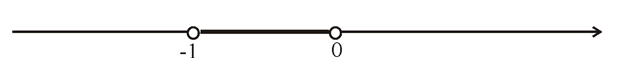# To graph: the given set.### Precalculus: Mathematics for Calcu...

6th Edition
Stewart + 5 others
Publisher: Cengage Learning
ISBN: 9780840068071### Precalculus: Mathematics for Calcu...

6th Edition
Stewart + 5 others
Publisher: Cengage Learning
ISBN: 9780840068071

#### Solutions

Chapter 1.1, Problem 60E
To determine

## To graph: the given set.

Expert Solution

### Explanation of Solution

Given information:

An interval is given as

(2,0)(1,1)

Concept used:

An interval (a,b) in terms of an inequality can be expressed as a<x<b .

An interval [a,b] in terms of an inequality can be expressed as axb .

An interval [a,b) in terms of an inequality can be expressed as ax<b .

An interval (a,b] in terms of an inequality can be expressed as a<xb .

An interval (,b] in terms of an inequality can be expressed as xb .

An interval (,b) in terms of an inequality can be expressed as x<b .

An interval [a,) in terms of an inequality can be expressed as xa .

An interval (a,) in terms of an inequality can be expressed as x>a .

For any two or more sets, intersection of the sets gives the elements which are the only common elements of the sets. Intersection is represented by the symbol .

Calculation:

Consider the given interval.

(2,0)(1,1)

Let us consider a variable x o express the inequality.

So, the interval can be expressed as an inequality as 2<x<01<x<1 .

Combining the interval will be 1<x<0=(1,0)

Graph:

Now, graph the interval on number line as shown:Here, 1and0 both the points are excluded from the interval so, open balls are placed for 1and0 on the number line.

### Have a homework question?

Subscribe to bartleby learn! Ask subject matter experts 30 homework questions each month. Plus, you’ll have access to millions of step-by-step textbook answers!